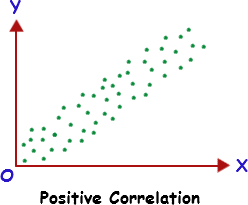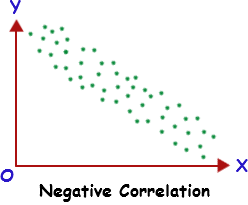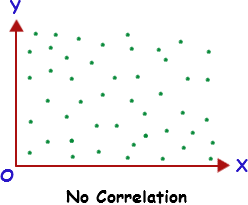The name correlation suggests the relationship between two variables as their Co-relation. The correlation coefficient is the measurement of correlation. To see how the two sets of data are connected, we make use of this formula. The linear dependency between the data set is done by the Pearson Correlation coefficient. It is also known as Pearson product moment correlation coefficient. The value of the Pearson correlation coefficient product is between -1 to +1.  When the correlation coefficient comes down to zero, then the data is said to be not related. While, if we are getting the value of +1, then the data are positively correlated and -1 has a negative correlation.

The graphical representation of positive, negative and no correlation is shown below:Positive CorrelationFor using the sample data, the Pearson correlation coefficient is denoted by the letter “r”. The formula for Pearson correlation coefficient r is given by:

r=n(xy)(x)(y)[nx2(x)2][ny2(y)2]

Where,
r = Pearson correlation coefficient
x = Values in the first set of data
y = Values in the second set of data
n = Total number of values.

Solved Examples

Question: Marks obtained by 5 students in algebra and trigonometry as given below:

 Algebra 15 16 12 10 8 Trigonometry 18 11 10 20 17

slide :- image only in phone  solve example ----------------------

Calculate the Pearson correlation coefficient?

Solution:

Construct the following table:

 x y x2 y2 xy 15 18 225 324 270 16 11 256 121 176 12 10 144 100 120 10 20 100 400 200 8 17 64 289 136 ∑x = 61 ∑y = 76 ∑x2 = 789 ∑y2 = 1234 ∑xy = 902

slide this image in only phone -------------------------------------------

Formula for Pearson correlation coefficient is given by:

r = n(xy)(x)(y)[nx2(x)2][ny2left(y)2]

r = 5×90261×76[5×789(61)2][5×1234(76)2]

r = -0.424

##### Alex robert

•